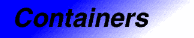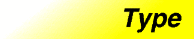# VectorCategory: containers Component type: type

## Description

A `vector` is a Sequence that supports random access to elements, constant time insertion and removal of elements at the end, and linear time insertion and removal of elements at the beginning or in the middle. The number of elements in a `vector` may vary dynamically; memory management is automatic. `Vector` is the simplest of the STL container classes, and in many cases the most efficient.

## Example

```vector<int> V;
V.insert(V.begin(), 3);
assert(V.size() == 1 && V.capacity() >= 1 && V == 3);
```

## Definition

Defined in the standard header vector, and in the nonstandard backward-compatibility header vector.h.

## Template parameters

Parameter Description Default
`T` The vector's value type: the type of object that is stored in the vector.
`Alloc` The `vector`'s allocator, used for all internal memory management. `Allocators`

## Type requirements

None, except for those imposed by the requirements of RandomAccessContainer and BackInsertionSequence.

None.

## Members

Member Where defined Description
`value_type` Container The type of object, `T`, stored in the vector.
`pointer` Container Pointer to `T`.
`reference` Container Reference to `T`
`const_reference` Container Const reference to `T`
`size_type` Container An unsigned integral type.
`difference_type` Container A signed integral type.
`iterator` Container Iterator used to iterate through a `vector`.
`const_iterator` Container Const iterator used to iterate through a `vector`.
`reverse_iterator` ReversibleContainer Iterator used to iterate backwards through a `vector`.
`const_reverse_iterator` ReversibleContainer Const iterator used to iterate backwards through a `vector`.
`iterator begin()` Container Returns an `iterator` pointing to the beginning of the `vector`.
`iterator end()` Container Returns an `iterator` pointing to the end of the `vector`.
`const_iterator begin() const` Container Returns a `const_iterator` pointing to the beginning of the `vector`.
`const_iterator end() const` Container Returns a `const_iterator` pointing to the end of the `vector`.
`reverse_iterator rbegin()` ReversibleContainer Returns a `reverse_iterator` pointing to the beginning of the reversed vector.
`reverse_iterator rend()` ReversibleContainer Returns a `reverse_iterator` pointing to the end of the reversed vector.
`const_reverse_iterator rbegin() const` ReversibleContainer Returns a `const_reverse_iterator` pointing to the beginning of the reversed vector.
`const_reverse_iterator rend() const` ReversibleContainer Returns a `const_reverse_iterator` pointing to the end of the reversed vector.
`size_type size() const` Container Returns the size of the `vector`.
`size_type max_size() const` Container Returns the largest possible size of the `vector`.
`size_type capacity() const` `vector` See below.
`bool empty() const` Container `true` if the `vector`'s size is `0`.
`reference operator[](size_type n)` RandomAccessContainer Returns the `n`'th element.
`const_reference operator[](size_type n) const` RandomAccessContainer Returns the `n`'th element.
`vector()` Container Creates an empty vector.
`vector(size_type n)` Sequence Creates a vector with `n` elements.
`vector(size_type n, const T& t)` Sequence Creates a vector with `n` copies of `t`.
`vector(const vector&)` Container The copy constructor.
```template <class InputIterator>
vector(InputIterator, InputIterator)
```

Sequence Creates a vector with a copy of a range.
`~vector()` Container The destructor.
`vector& operator=(const vector&)` Container The assignment operator
`void reserve(size_t)` `vector` See below.
`reference front()` Sequence Returns the first element.
`const_reference front() const` Sequence Returns the first element.
`reference back()` BackInsertionSequence Returns the last element.
`const_reference back() const` BackInsertionSequence Returns the last element.
`void push_back(const T&)` BackInsertionSequence Inserts a new element at the end.
`void pop_back()` BackInsertionSequence Removes the last element.
`void swap(vector&)` Container Swaps the contents of two vectors.
```iterator insert(iterator pos,
const T& x)
```
Sequence Inserts `x` before `pos`.
```template <class InputIterator>
void insert(iterator pos,
InputIterator f, InputIterator l)
```

Sequence Inserts the range `[first, last)` before `pos`.
```void insert(iterator pos,
size_type n, const T& x)
```
Sequence Inserts `n` copies of `x` before `pos`.
`iterator erase(iterator pos)` Sequence Erases the element at position `pos`.
`iterator erase(iterator first, iterator last)` Sequence Erases the range `[first, last)`
`void clear()` Sequence Erases all of the elements.
`void resize(n, t = T())` Sequence Inserts or erases elements at the end such that the size becomes `n`.
```bool operator==(const vector&,
const vector&)
```
ForwardContainer Tests two vectors for equality. This is a global function, not a member function.
```bool operator<(const vector&,
const vector&)
```
ForwardContainer Lexicographical comparison. This is a global function, not a member function.

## New members

These members are not defined in the RandomAccessContainer and BackInsertionSequence requirements, but are specific to `vector`.

Member Description
`size_type capacity() const` Number of elements for which memory has been allocated. `capacity()` is always greater than or equal to `size()`.  
`void reserve(size_type n)` If `n` is less than or equal to `capacity()`, this call has no effect. Otherwise, it is a request for allocation of additional memory. If the request is successful, then `capacity()` is greater than or equal to `n`; otherwise, `capacity()` is unchanged. In either case, `size()` is unchanged.